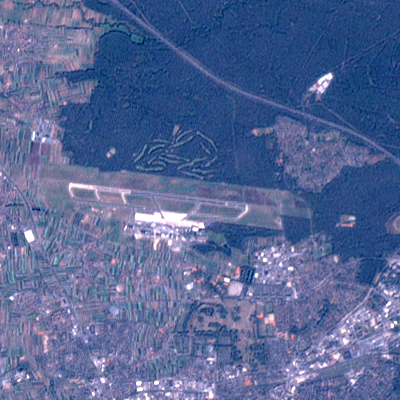LibGrid

## Pansharpening / Linear Regression

Pansharpening a la Linear Regression:

$Pan = \alpha R + \beta G + \gamma B + \theta NIR + c$
• Determine $\alpha, \beta, \gamma, \theta$ und $c$ by multiple linear regression
• Then $V' = Pan \cdot \frac{V}{\alpha R + \beta G + \gamma B + \theta NIR + c}$
• Brovey is the special case for $\alpha=\beta=\gamma=\frac13$, $\theta=c=0$.

For more information about pansharpening see the GRASS GIS manual. Note that GRASS offers a PCA (principal component analysis) to perform the pansharpening, but in my opinion the PCA alters the original colors too much, while linear regression just alters the intensities and keeps the original colors as much as possible.RGB combined with linear regression, 15m